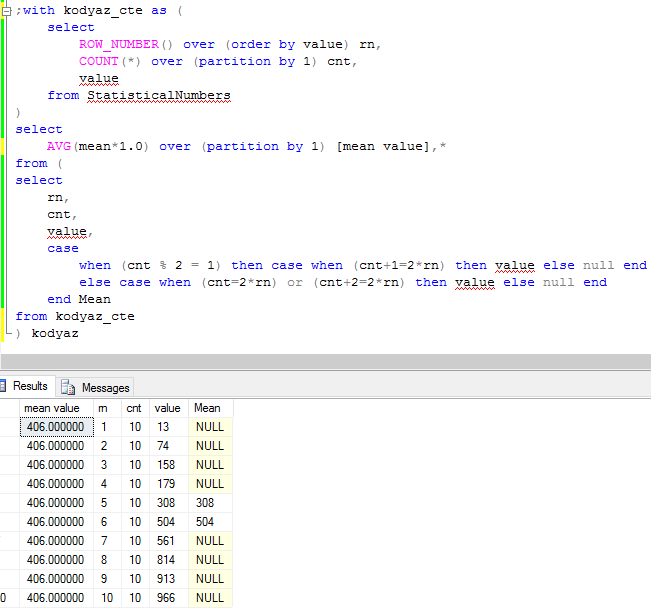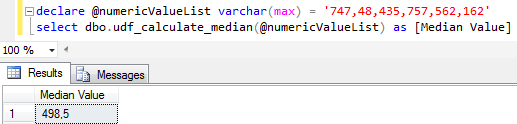SQL Server and T-SQL Development Tutorials Development resources, articles, tutorials, code samples and tools and downloads for ASP.Net, SQL Server, R Script, Windows, Windows Phone, AWS, SAP HANA and ABAP, like SAP UI5, Screen Personas, etc.
 Home Articles News IT Jobs Tools Sample Chapters Trainers Blogs Forums Photos Files

SQL Server 2019 Installation

# Calculate Median Value of a Numeric List in SQL Server

SQL programmers can calculate median value of a numeric array in SQL Server by using Row_Number(), Count() and other SQL Server aggregate functions. Transact-SQL developers or database administrators can use median value calculation method on any numeric table column in a SQL Server database as well as the can calculate the median value of a given list as an input parameter to the SQL median function.

Let's create a database table to store numerical values like an array of numeric values. This value table column can be used for statistical values entry and storage by SQL developers.

``` create table StatisticalNumbers(  id int identity(1,1),  value int ) ```

Now, let SQL developers or database administrators to generate sample data and insert into our SQL database table for the calculation of median value of numbers column. I used SQL RAND() function to generate random numbers between 0 and 1. By multiplying the random value with 100, I have random values between 0 and 99 for sample test data.

``` declare @i int = 1 while @i <= 100 begin  insert into StatisticalNumbers SELECT RAND()*100  set @i=@i+1 end ```

Here is the source codes of the SQL script which can be used to calculate the median value of a numeric value list given on SQL Server.

SQL Server CTE expression counts the numeric values in the sample array and sorts the numeric values by using SQL Row_Number() function in an ascending way.

Statistical median value calculation is done by first marking the values at the middle of the array when the array items are sorted ascending. If the count of the numerical list items is an "odd" number then the value is easily read from the list. On the other hand, if the number or numeric values in the sample array is "even", then the two values that are at the middle are summed and then divided by two.

``` ;with kodyaz_cte as (  select   ROW_NUMBER() over (order by value) rn,   COUNT(*) over (partition by 1) cnt,   value  from StatisticalNumbers ) select  AVG(Median*1.0) over (partition by 1) [median value],* from ( select  rn,  cnt,  value,  case   when (cnt % 2 = 1) then case when (cnt+1=2*rn) then value else null end   else case when (cnt=2*rn) or (cnt+2=2*rn) then value else null end  end Median from kodyaz_cte ) kodyaz ```

Here is a screenshot of the above median calculation in SQL Server.Calculate median value of an array in SQL Server

It is also possible to create a user defined SQL function which takes the numeric values as a string input parameter formed of concatenated numeric values and returns the median value as follows.

Please note that a second SQL function is used to split concatenated numeric values using SQL named dbo.split(). SQL Server developers can find the source codes of the SQL Split function in the reference SQL tutorial.

``` create function udf_calculate_median (  @numericValueList varchar(max) ) returns float as begin declare @median float ;with kodyaz_cte as (  select   ROW_NUMBER() over (order by value) rn,   COUNT(*) over (partition by 1) cnt,   value  from (   select CAST(val as int) value   from dbo.split(@numericValueList,',')  ) StatisticalNumbers ) select  @median = AVG(Median*1.0) over (partition by 1) from ( select  rn,  cnt,  value,  case   when (cnt % 2 = 1) then case when (cnt+1=2*rn) then value else null end   else case when (cnt=2*rn) or (cnt+2=2*rn) then value else null end  end Median from kodyaz_cte ) kodyaz  return @median end ```

And this sample SQL function can be used to calculate median value of a numeric list as given in the following example on SQL Server

``` declare @numericValueList varchar(max) = '747,48,435,757,562,162' select dbo.udf_calculate_median(@numericValueList) as [Median Value] ```Median value calculation function for SQL Server developers

Related SQL Resources

SQL Server Articles

SQL Server 2012

SQL Server Tools

SQL Blog

MS SQL Server Forums